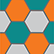Online Program

Saturday, May 19Bioinformatics
Sat, May 19, 10:30 AM - 12:00 PM
Regency Ballroom A

Scalable and Flexible Probabilistic PCA for Large-Scale Genetic Variation Data (304450)

Aman Agrawal, IIT Delhi
Eran Halperin, UCLA
Minh Le, UCLA
*Sriram Sankararaman, UCLA

Keywords: Latent factor models, PCA, EM, Genetic variation

Principal Component Analysis (PCA) is a key tool in population and medical genetic studies. With the advent of large-scale datasets of genetic variation, there is a need for methods that can compute principal components with scalable computational and memory requirements. Current exact methods for computing principal components do not scale to large datasets.

We propose a scalable and exact algorithm to compute principal components on genetic variation data. Our method is based on a previously proposed latent variable model for probabilistic PCA, PPCA, of which PCA arises in the small variance limit. The latent variable model formulation leads to an iterative EM algorithm for computing the principal components with time complexity O(NMK) to compute K principal components on N individuals and M SNPs per iteration. The key computational bottleneck in the EM algorithm consists of multiplying the genotype matrix with a small number of real-valued vectors. Leveraging the structure of the genotype matrix, we can perform a O(NM) pre-processing of this matrix so that the per-iteration time complexity reduces to O(NMK/(max?(log?N,log?M))) . These two ideas lead to a highly-scalable, exact algorithm for PCA.

We show the accuracy and scalability of our method on simulations. We show that our method can compute the top ten PCs on a dataset of 500,000 individuals and 100,000 SNPs in less than an hour on standard hardware.

The probabilistic generative formulation also allows the model to be generalized in a number of ways. One direction that we have explored is the application of PCA in the presence of missing and we show that the probabilistic model leads to more accurate estimates of PCs.